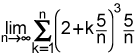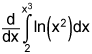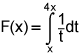# 8 short answer calc questions

## 8 short answer calc questions

I’m stuck on a Calculus question and need an explanation.

1.

Writeas a definite integral. (10 points)

2.

Find. (10 points)

3.

Find the area bounded by the curves y2 = 2x + 6 and x = y + 1. Your work must include an integral in one variable. (10 points)

4.

Write the integral in one variable to find the volume of the solid obtained by rotating the first-quadrant region bounded by y = 0.5x2 and y = x about the line x = 3. (10 points)

5.

A rock is thrown upward with a speed of 48 feet per second from the edge of a cliff 400 feet above the ground. What is the speed of the rock when it hits the ground? Use acceleration due to gravity as -32 feet per second squared and approximate your answer to 3 decimal places. (10 points)

6.

Use the mid-point rule with n = 4 to approximate the area of the region bounded by y = x3 and y = x. (10 points)

7.

Electricity is consumed between the hours of midnight (t = 0) and 6 a.m. (t = 6). Selected values of the rate of consumption are shown in the table below, with t measured in hours past midnight, and R(t) measured in KW per hour. Use 4 trapezoids to estimate the total amount of electricity to the nearest KW consumed between midnight and 6 a.m. (10 points)

t (hours) 0 1 2 5 6
R(t) KW/hr 400 200 350 200 150

8.

Show that the functionis constant on the interval (0, +∞). (10 points)